# Objects in Memory

We often talk about the class as a blueprint for an object. This is because classes define what properties and methods an object should have, in the form of the class definition. An object is created from this blueprint by invoking the class’ constructor. Consider this class representing a planet:

``````/// <summary>
/// A class representing a planet
// </summary>
public class Planet {

/// <summary>
/// The planet's mass in Earth Mass units (~5.9722 x 10^24kg)
/// </summary>
private double mass;
public double Mass
{
get { return mass; }
}

/// <summary>
/// </summary>
{
}

/// <summary>
/// Constructs a new planet
/// <param name="mass">The planet's mass</param>
{
this.mass = mass;
}

}
``````

It describes a planet as having a mass and a radius. But a class does more than just labeling the properties and fields and providing methods to mutate the state they contain. It also specifies how memory needs to be allocated to hold those values as the program runs. In memory, we would need to hold both the mass and radius values. These are stored side-by-side, as a series of bits that are on or off. You probably remember from CIS 115 that a `double` is stored as a sign bit, mantissa and exponent. This is also the case here - a C# `double` requires 64 bits to hold these three parts, and we can represent it with a memory diagram: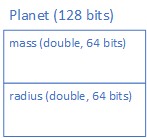We can create a specific planet by invoking its constructor, i.e.:

``````new Planet(1, 1);
``````

This allocates (sets aside) the memory to hold the planet, and populates the mass and radius with the supplied values. We can represent this with a memory diagram: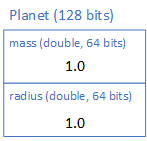With memory diagrams, we typically write the values of variables in their human-readable form. Technically the values we are storing are in binary, and would each be `0000000000010000000000000000000000000000000000000000000000000001`, so our overall object would be the bits: `00000000000100000000000000000000000000000000000000000000000000010000000000010000000000000000000000000000000000000000000000000001`.

And this is exactly how it is stored in memory! The nice boxes we drew in our memory diagram are a tool for us to reason about the memory, not something that actually exists in memory. Instead, the compiler determines the starting point for each `double` by looking at the structure defined in our `class`, i.e. the first field defined is `mass`, so it will be the first 64 bits of the object in memory. The second field is `radius`, so it starts 65 bits into the object and consists of the next (and final) 64 bits.

If we assign the created `Planet` object to a variable, we allocate memory for that variable:

``````Planet earth = new Planet(1, 1);
``````

Unlike our `double` and other primitive values, this allocated memory holds a reference (an starting address of the memory where the object was allocated). We indicate this with a box and arrow connecting the variable and object in our memory diagram: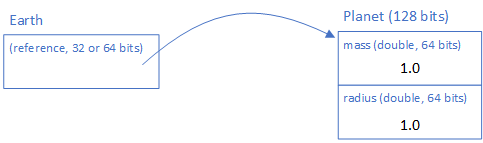A reference is either 32 bits (on a computer with a 32-bit CPU) or 64 bits (on a computer with a 64-bit CPU), and essentially is an offset from the memory address \$0\$ indicating where the object will be located in memory (in the computer’s RAM). You’ll see this in far more detail in CIS 450 - Computer Architecture and Operations, but the important idea for now is the variable stores where the object is located in memory not the object’s data itself. This is also why if we define a class variable but don’t assign it an object, i.e.:

``````Planet mars;
``````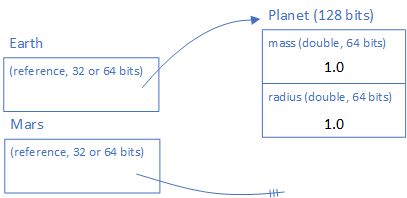The value of this variable will be `null`. It’s because it doesn’t point anywhere!

Returning to our Earth example, `earth` is an instance of the class `Planet`. We can create other instances, i.e.

``````Planet mars = new Planet(0.107, 0.53);
``````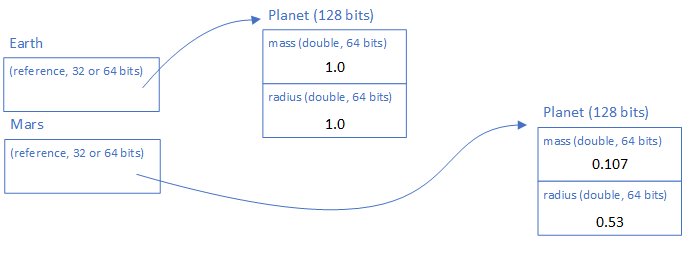We can even create a Planet instance to represent one of the exoplanets discovered by NASA’s TESS:

``````Planet hd21749b = new Planet(23.20, 2.836);
``````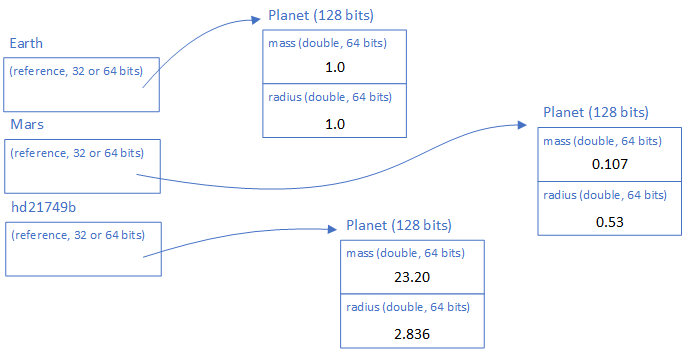Let’s think more deeply about the idea of a class as a blueprint. A blueprint for what, exactly? For one thing, it serves to describe the state of the object, and helps us label that state. If we were to check our variable mars’ radius, we do so based on the property Radius defined in our class:

``````mars.Radius
``````

This would follow the `mars` reference to the `Planet` object it represents, and access the second group of 64 bits stored there, interpreting them as a `double` (basically it adds 64 to the `reference` address and then reads the next 64 bits)

State and memory are clearly related - the current state is what data is stored in memory. It is possible to take that memory’s current state, write it to persistent storage (like the hard drive), and then read it back out at a later point in time and restore the program to exactly the state we left it with. This is actually what Windows does when you put it into hibernation mode.

The process of writing out the state is known as serialization, and it’s a topic we’ll revisit later.